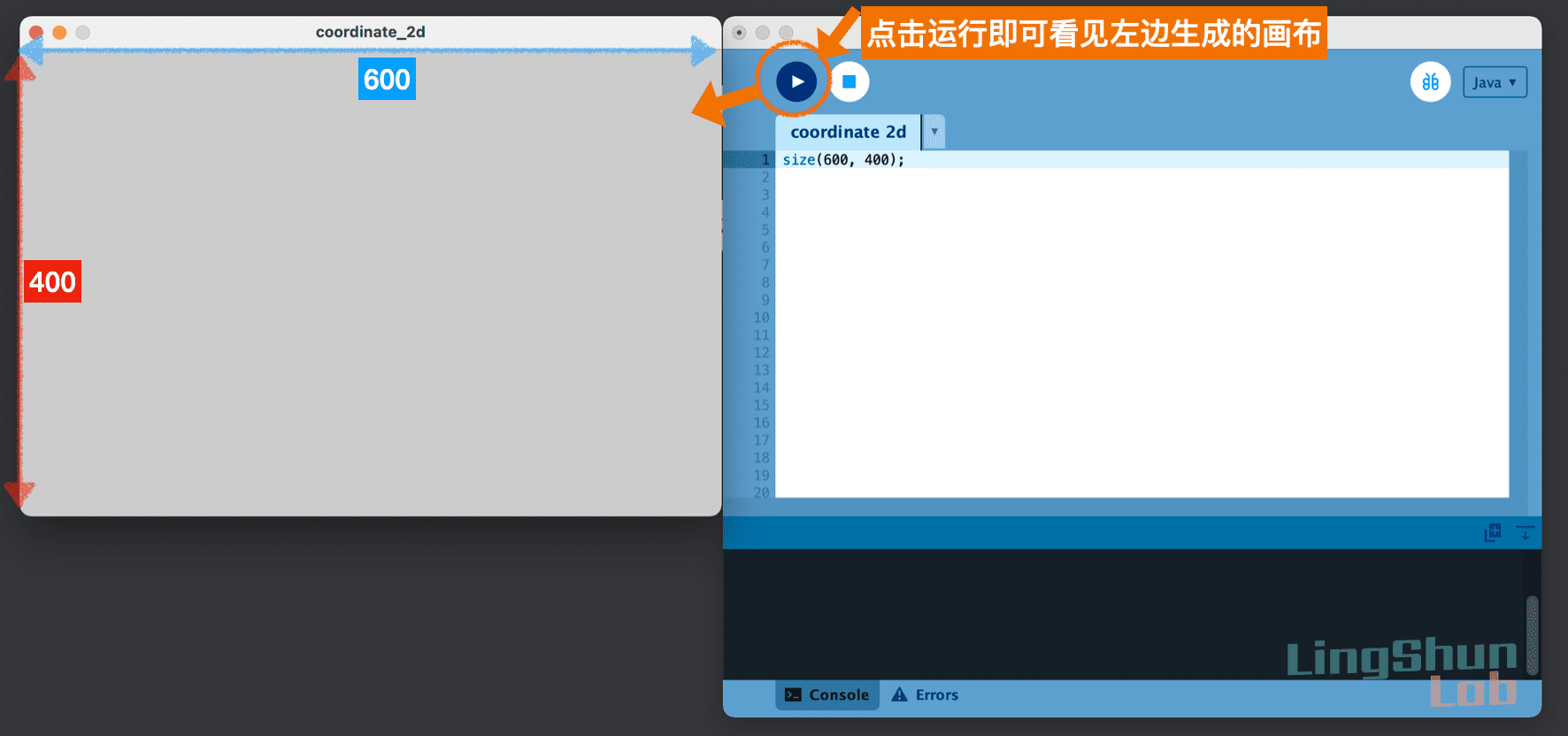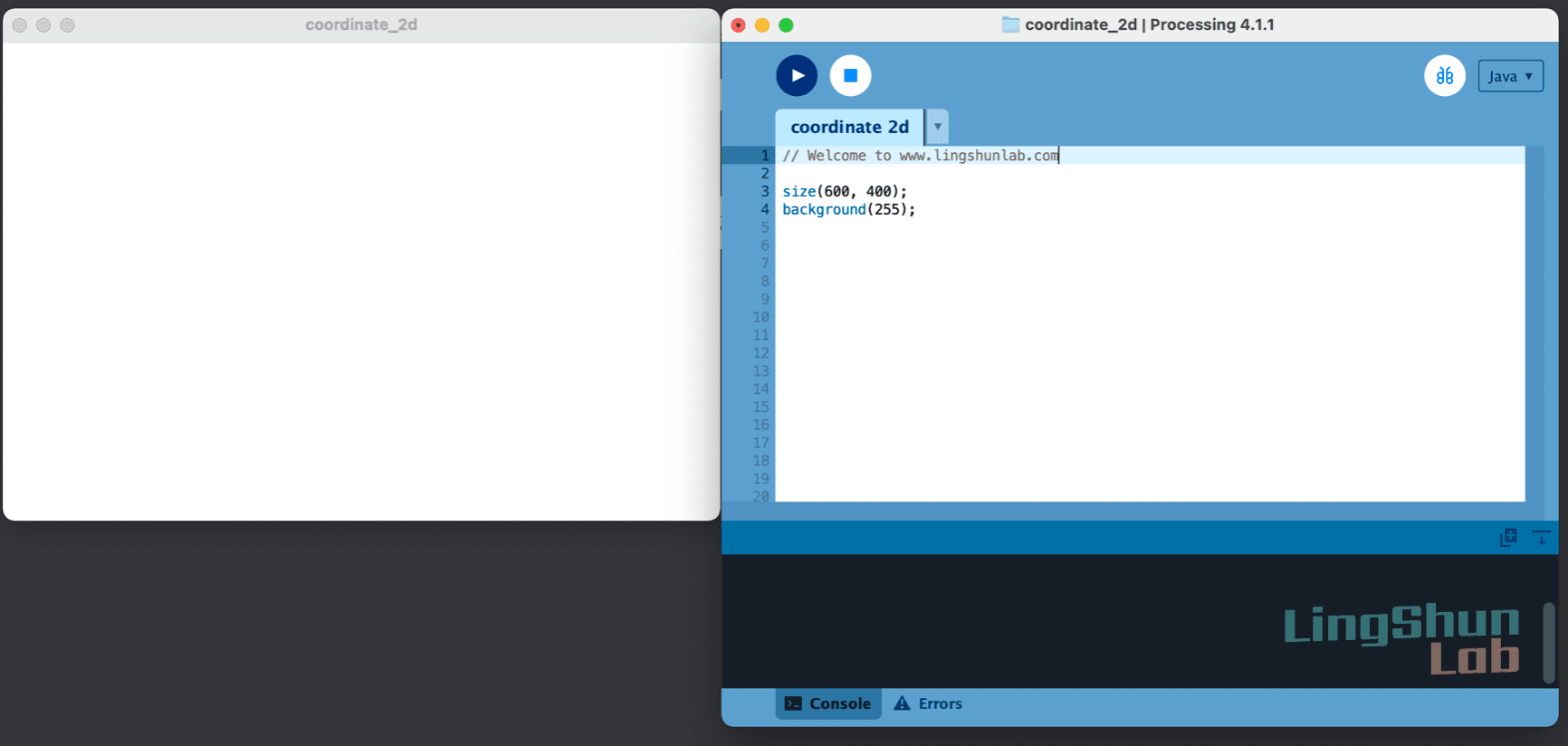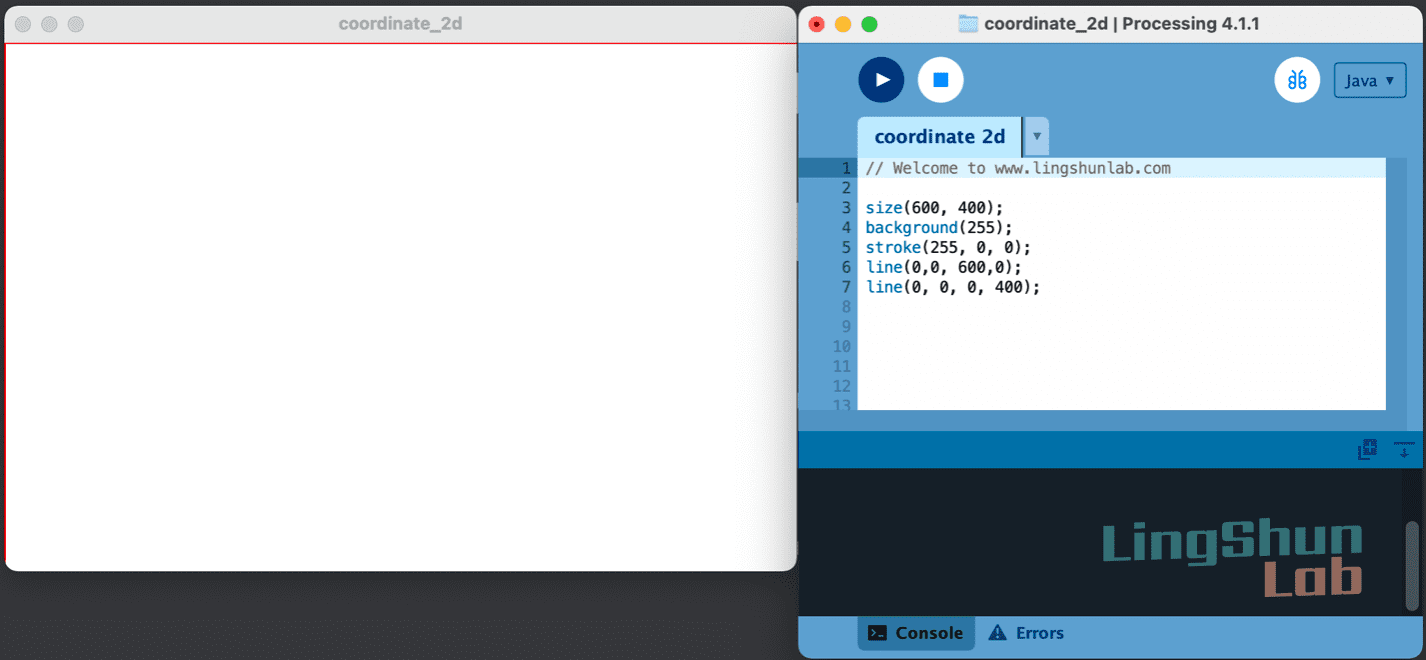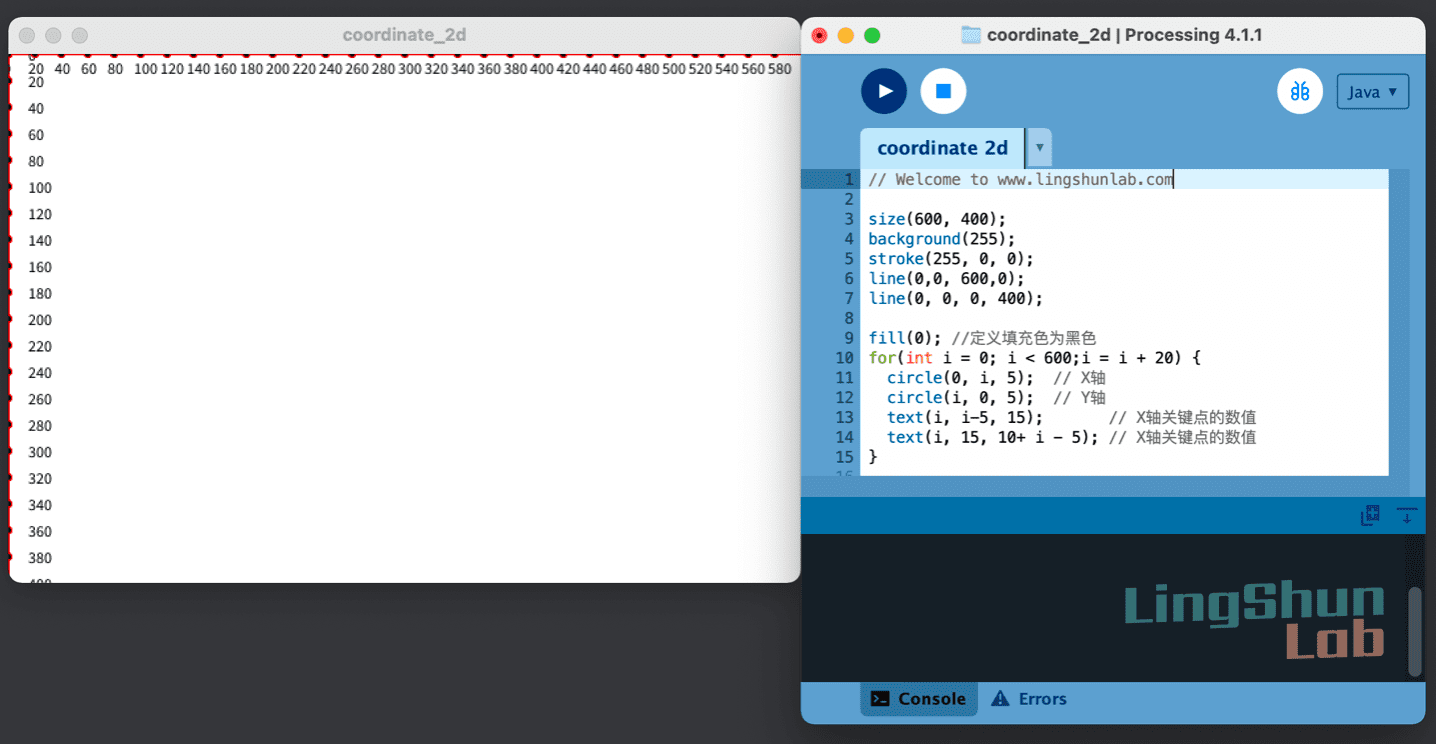# Processing 的2D坐标

## 定义画布

size(600,400)中的第一个参数是是定义画布的宽度，也是X轴的长度，第二个参数 定义的是 画布的高度，也就是 Y周的长度。在Processing中最小的画布限制在宽100像素，高100像素中，如果设置小于此像素的画布会强制设定为100x100。size() 官方详细说明请点击
https://processing.org/reference/size_.html

## 设定背景颜色

size(600, 400);
background(255);background() 官方详细说明请点击
https://processing.org/reference/background_.html

## X轴上，画一条线

size(600, 400);
background(255);
line(0,0, 600,0);line() 官方详细说明请点击
https://processing.org/reference/line_.html

## Y轴上，画一条线

// Welcome to www.lingshunlab.com

size(600, 400);
background(255);
line(0,0, 600,0);
line(0, 0, 0, 400);## 设置线段的颜色

stroke(255, 0, 0) 函数有三个参数，

// Welcome to www.lingshunlab.com

size(600, 400);
background(255);
stroke(255, 0, 0);
line(0,0, 600,0);
line(0, 0, 0, 400);stroke() 官方详细说明请点击
https://processing.org/reference/stroke
.html

## XY轴上，画一些圆形标记

// 标记X轴的关键位置
circle(0, 20, 5);
circle(0, 40, 5);
circle(0, 60, 5);
circle(0, 80, 5);
...

// 标记Y轴的关键位置
circle(20, 0, 5);
circle(40, 0, 5);
circle(60, 0, 5);
circle(80, 0, 5);
...


for(int i = 0; i < 600;i = i + 20) {
circle(0, i, 5);
circle(i, 0, 5);
}

// Welcome to www.lingshunlab.com

size(600, 400);
background(255);
stroke(255, 0, 0);
line(0,0, 600,0);
line(0, 0, 0, 400);

for(int i = 0; i < 600;i = i + 20) {
circle(0, i, 5);  // 标记X轴的关键位置
circle(i, 0, 5);  // 标记Y轴的关键位置
}for语句 官方详细说明请点击
https://processing.org/reference/stroke_.html

## 关键点添加数字

fill(0);有一个参数，指定介于白色和黑色之间的值，0为黑色，255为白色。

fill(0);函数和stroke()函数类似，前者是设置填充色，后者是设置边线颜色。都需要在绘画前就定义好，

// Welcome to www.lingshunlab.com

size(600, 400);
background(255);
stroke(255, 0, 0);
line(0,0, 600,0);
line(0, 0, 0, 400);

fill(0); //定义填充色为黑色
for(int i = 0; i < 600;i = i + 20) {
circle(0, i, 5);  // X轴
circle(i, 0, 5);  // Y轴
text(i, i-5, 15);       // X轴关键点的数值
text(i, 15, 10+ i - 5); // X轴关键点的数值
}fill() 官方详细说明请点击
https://processing.org/reference/fill_.html
text() 官方详细说明请点击
https://processing.org/reference/text_.html

## 显示当前鼠标的坐标

// 初始化函数
void setup(){
...
//setup内可以放置程式启动时要做的事情，例如是设定背景颜色或画布大小。
//setup只运行一次。
}

void draw() {
...
//draw内的代码则是每次画面更新要做什麽事情，像是各种绘制的变化等等
//draw会不断重复运行里面的代码，如果玩过Arduino 就感觉这不就是loop()函数吗
}

    // Welcome to www.lingshunlab.com
void  setup() {  // // 初始化
size(600, 400);
}

void draw() { // 重复
background(255);
stroke(255, 0, 0);
line(0,0, 600,0);
line(0, 0, 0, 400);

fill(0); //定义填充色为黑色
for(int i = 0; i < 600;i = i + 20) {
circle(0, i, 5);  // X轴
circle(i, 0, 5);  // Y轴
text(i, i-5, 15);       // X轴关键点的数值
text(i, 15, 10+ i - 5); // X轴关键点的数值
}
}

circle(mouseX, mouseY, 2);
// Welcome to www.lingshunlab.com
void  setup() {
size(600, 400);
}

void draw() {
clear();
background(255);
stroke(255, 0, 0);
line(0,0, 600,0);
line(0, 0, 0, 400);

fill(0); //定义填充色为黑色
for(int i = 0; i < 600;i = i + 20) {
circle(0, i, 5);  // X轴
circle(i, 0, 5);  // Y轴
text(i, i-5, 15);       // X轴关键点的数值
text(i, 15, 10+ i - 5); // X轴关键点的数值
}

//文字方式显示鼠标当前的坐标数值
text(" ( "+ str(mouseX)+ ", " + str(mouseY)+ " )", mouseX+5, mouseY+5);
//在鼠标当前坐标上画一个小圆
circle(mouseX, mouseY, 2);
}